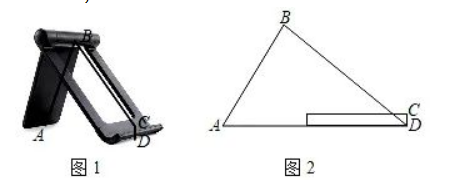. 如图 1 是一手机支架，其中 $A B=8 \mathrm{~cm}$, 底麼 $C D=1 \mathrm{~cm}$, 当点 $A$ 正好落在桌面上时如图2所示, $\angle A B C=$ $80^{\circ}, \angle A=60^{\circ}$.
(1) 求点 $B$ 到桌面 $A D$ 的距离;
(2) 求 $B C$ 的长. (结果精确到 $0.1 \mathrm{~cm}$; 参考数据: $\sin 50^{\circ} \approx 0.77, \cos 50^{\circ} \approx 0.64, \tan 50^{\circ} \approx 1.19$, $\sqrt{3} \approx 1.73)$